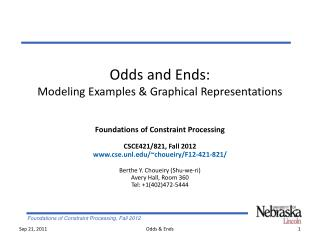DownloadDownload PresentationOdds and Ends: Modeling Examples & Graphical Representations

# Odds and Ends: Modeling Examples & Graphical Representations

Télécharger la présentation## Odds and Ends: Modeling Examples & Graphical Representations

- - - - - - - - - - - - - - - - - - - - - - - - - - - E N D - - - - - - - - - - - - - - - - - - - - - - - - - - -
##### Presentation Transcript

1. Odds and Ends:Modeling Examples & Graphical Representations Foundations of Constraint Processing CSCE421/821, Fall 2012 www.cse.unl.edu/~choueiry/F12-421-821/ Berthe Y. Choueiry (Shu-we-ri) Avery Hall, Room 360 Tel: +1(402)472-5444 Odds & Ends

2. Outline • Modeling examples • Minesweeper, Game of Set • Graphical representations Odds & Ends

3. Minesweeper • Variables? • Domains? • Constraints? Odds & Ends

4. Minesweeper as a CSP demo • Variables are the cells • Domains are {0,1} (i.e., safe or mined) • One constraint for each cell with a number (arity 1...8) Exactly two mines: 0000011 0000101 0000110, etc. Exactly three mines: 0000111 0001101 0001110, etc. Joint work with R. Woodward, K. Bayer & J. Snyder Odds & Ends

5. Game of Set [Falco 74] • Deck of 81(=34) cards, each card with a unique combination of 4 attributes values • Number  {1,2,3} • Color {green,purple,red} • Filling  {empty,stripes, full} • Shape  {diamond,squiggle,oval} • Solution set: 3 cards  attribute, the 3 cards have either the same value or all different values • 12 cards are dealt, on table [3,21] • Recreational game, favorite of children & CS/Math students • New toy problem for AI: a typical multi-dimensional CSP Joint work with Amanda Swearngin and Eugene C. Freuder Odds & Ends

6. Set: Constraint Model I c2 c3 c1 • Model I • Three variables • Same domain (12 cards) • One ‘physical’ constraints • Four 1-dimensional constraints • Size of model? c6 c5 c4 c8 c9 c7 id≠ c1,c2,c3,…,c12 S=⊕S≠ C=⊕C≠ c1,c2,c3,…,c12 F=⊕F≠ N=⊕N≠ c1,c2,c3,…,c12 Odds & Ends

7. Set: Constraint Model II • Model II • 12 variables (as many as on table) • Boolean domains {0,1} • Constraints: much harder to express • Exactly 3 cards: Sum(assigned values)=3? • AllEqual/AllDiff constraints? • Size of model? Odds & Ends

8. Graphical Representations • Always specify V,E for a graph as G=(V,E) • Main representations • Binary CSPs • Graph (for binary CSPs) • Microstructure (supports) • Co-microstructure (conflicts) • Non-binary CSPs • Hypergraph • Primal graph • Dual graph Odds & Ends

9. V1 a, b   V3 V2 a, c b, c  Binary CSPs Macrostructure G(P)=(V,E) • V= • E= Micro-structure(P)=(V,E) • V= • E= Co-microstructure co-(P)=(V,E) • V= • E= Supports (V1, a ) (V1, b) (V2, a ) (V2, c) (V3, b ) (V3, c) No goods (V1, a ) (V1, b) (V2, a ) (V2, c) (V3, b ) (V3, c) Odds & Ends

10. Non-binary CSPs: Hypergraph • Hypergraph (non-binary CSP) • V= • E= R4 R6 R6 B A A B R4 E R2 R5 E D R1 R2 R5 R3 R1 R3 C F F C D Odds & Ends

11. Non-binary CSPs: Primal Graph • Primal graph • V= • E= R6 B A B A R4 E E R1 R2 R5 R3 C F D F D C Odds & Ends

12. Dual Graph R6 B A • V= • G= R4 E R1 R2 R5 R3 C F D R5 R3 R1 C D AD BCD CF A B BD AD F AB ABDE EF AB E R6 R4 R2 Hypergraph Dual graph Odds & Ends